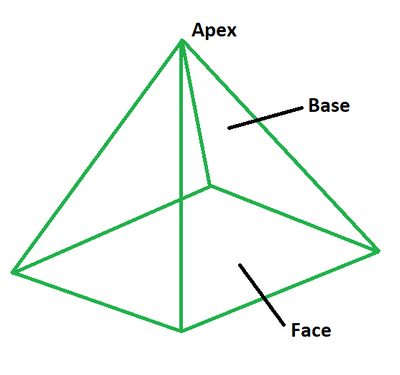# How to find the Surface Area of a Square Pyramid?

• Last Updated : 23 May, 2022

A pyramid is defined as a three-dimensional polyhedron with three or more triangle-shaped faces that meet above the base and a polygonal base. The triangle sides are the faces, while the apex is the point above the base. To construct a pyramid, the base is connected to the summit. The pyramid is known as a square pyramid when its base is square. A square pyramid has three triangular sides and a square base. In other terms, it has 8 edges, 5 vertices, and 4 faces.### Total Surface Area Formula of Square Pyramid

A square pyramid’s total surface area is equal to the total area covered by the four triangular sides and a square base. Its formula is equal to the sum of the base area and twice the product of base length and slant height.

TSA = a2 + 2al

where,

• TSA is the total surface area,
• a is the base length,
• l is the slant height.

In terms of base length and height of the pyramid, the formula is expressed as:

TSA = a2 + 2a √(a2/4 + h2)

where,

• TSA is the total surface area,
• a is the base length,
• h is the height or altitude.

### Lateral Surface Area Formula of Square Pyramid

A square pyramid’s lateral surface area is defined as the area covered by its four triangular faces. Its formula is equal to twice the product of base length and slant height. It can be interpreted as the total surface area reduced by the base area of a square pyramid.

LSA = 2al

where,

• LSA is the total surface area,
• a is the base length,
• l is the slant height.

In terms of base length and height of the pyramid, the formula is expressed as:

LSA = 2a √(a2/4 + h2)

where,

• LSA is the lateral surface area,
• a is the base length,
• h is the height or altitude.

### Sample Problems

Problem 1: Calculate the total surface area of a square pyramid if its base is 10 cm and slant height is 13 cm.

Solution:

We have,

a = 10

l = 13

Using the formula we have,

TSA = a2 + 2al

= (10 × 10) + (2 × 10 × 13)

= 100 + 260

= 360 sq. cm

Problem 2: Calculate the total surface area of a square pyramid if its base is 6 cm and slant height is 8.54 cm.

Solution:

We have,

a = 6

l = 8.54

Using the formula we have,

TSA = a2 + 2al

= (6 × 6) + (2 × 6 × 8.54)

= 36 + 102.53

= 138.53 sq. cm

Problem 3: Calculate the total surface area of a square pyramid if its base is 11 cm and height is 9 cm.

Solution:

We have,

a = 11

h = 9

Using the formula we have,

TSA = a2 + 2a√(a2/4 + h2)

= (11 × 11) + (2 × 11 √(112/4 + 92))

= 121 + 232.05

= 353.05 sq. cm

Problem 4: Calculate the total surface area of a square pyramid if its base is 14 cm and height is 10 cm.

Solution:

We have,

a = 14

h = 10

Using the formula we have,

TSA = a2 + 2a√(a2/4 + h2)

= (14 × 14) + (2 × 14 √(142/4 + 102))

= 196 + 341.8

= 537.8 sq. cm

Problem 5: Calculate the lateral surface area of a square pyramid if its base is 3 cm and slant height is 4.27 cm.

Solution:

We have,

a = 3

l = 4.27

Using the formula we have,

LSA = 2al

= 2 × 13 × 4.27

= 25.63 sq. cm

Problem 6: Calculate the lateral surface area of a square pyramid if its base is 13 cm and height is 10 cm.

Solution:

We have,

a = 13

h = 10

Using the formula we have,

LSA = 2a√(a2/4 + h2)

= 2 × 13 √(132/4 + 102)

= 310.1 sq. cm

Problem 7: Calculate the lateral surface area of a square pyramid if its base is 9 cm and height is 14 cm.

Solution:

We have,

a = 9

h = 14

Using the formula we have,

LSA = 2a√(a2/4 + h2)

= 2 × 9 √(92/4 + 142)

= 264.7 sq. cm

My Personal Notes arrow_drop_up
Recommended Articles
Page :